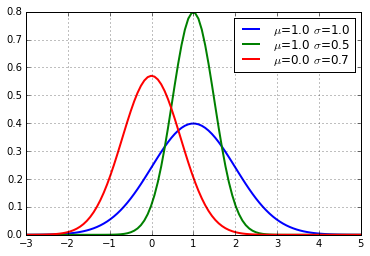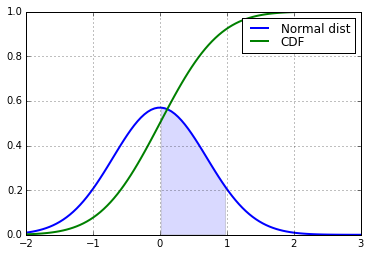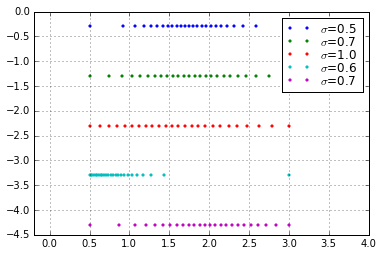# Generating a number range with normal spacing density¶

We want to generate N numbers between x_min and x_max with both limits included. This is trivial to do with a uniform distribution, for example with np.linspace(x_min, x_max, N) in NumPy.

But sometimes it can be helpful to have a range of numbers that are more concentrated around a specific area. For example if they are used as input for monte-carlo simulations. Ideally their density would be proportional to a normal distribution. That shall be the goal of this notebook! Source is on github.

We'll assume a normal definition with a standard deviation of $\sigma$ and a center of $\mu$:

$$f(x) = \frac{1}{\sigma \sqrt{2\pi}}\exp\left(-\frac{(x-\mu)^2}{2\sigma^2}\right)$$

Let's have a look:

In :
import numpy as np
import matplotlib as mpl
import matplotlib.pyplot as plt
from scipy.stats import norm
%matplotlib inline
mpl.rcParams['lines.linewidth'] = 2
mpl.rcParams['axes.grid'] = True
mpl.rcParams['legend.labelspacing'] = 0.0

In :
x = np.linspace(-3, 5, 100)
parameters = [(1.0, 1.0), (1.0, 0.5), (0.0, 0.7)]
for mu, sigma in parameters:
plt.plot(x, norm.pdf(x, mu, sigma), label="$\\mu$={} $\\sigma$={}".format(mu, sigma))
plt.legend();The key idea for our range is that the points divide a normal distribution into segments of equal areas. So first we need to look at the integrals. Normal distributions are always normalized, so $\displaystyle\int_{-\infty}^\infty f(x) dx=1$. The integral up to a certain point is given by the cumulative distribution function $\displaystyle\text{cdf}(x)=\int_{-\infty}^x f(x) dx$. For the normal distrubution, that is

$$\text{cdf}(x) = \frac 1 2 \left(1+\text{erf}\left(\frac{x-\mu}{\sigma\sqrt 2}\right)\right)$$

Let's have a look:

In :
x = np.linspace(-2, 3, 100)
x_min=0.0; x_max=1.0; mu0=0.0; sigma0=0.7
plt.plot(x, norm.pdf(x, mu0, sigma0), label="Normal dist")
plt.fill_between(x, 0, norm.pdf(x, mu0, sigma0), where=(x_max>x)&(x>x_min), alpha=0.15)
plt.plot(x, norm.cdf(x, mu0, sigma0), label="CDF")
plt.legend();We want to look at the case where $f(x)$ is only defined on some interval $[x_\text{min}, x_\text{max}]$, that's hinted by the shaded area. That area is $A=\text{cdf}(x_\text{max})-\text{cdf}(x_\text{min})$. So the total integral is divided into $A$ + the area left of it ($\text{cdf}(x_\text{min})$) + the area right of it (not important). Since we know all areas, we'll solve $\text{cdf}(x)$ for $x$:

$$x=\sigma\sqrt 2\cdot\text{erf}^{-1}(2\cdot\text{cdf}(x)-1)+\mu$$

To generate our desired range, we'll evaluate that equation for different values of cdf(x). We have to start at $\text{cdf}(x_\text{min})$ and step through $N-1$ parts of $A$. So we divide $A$ into $A_0=\frac{A}{N-1}$ and can write down the result. The cases i=0 and i=N-1 are identical to x_min and x_max.

Technical note: SciPy has the inverse error function and the pdf included. In C++ we need Boost.

In :
from scipy.special import erfinv

def normalSpace(x_min, x_max, N, mu, sigma):
area_min = norm.cdf(x_min, mu, sigma)
area_max = norm.cdf(x_max, mu, sigma)
A = area_max - area_min
A0 = A/(N-1)
seq = [x_min] + [np.sqrt(2)*sigma*erfinv((area_min+i*A0)*2-1)+mu for i in range(1,N-1)] + [x_max]
return np.asarray(seq)

fig = plt.subplot(xlim=(-0.2,4))
parameters = [(1.75, 0.5), (1.75, 0.7), (1.5, 1.0), (0.0, 0.6), (2.0, 0.7)]
for i, (mu, sigma) in enumerate(parameters):
x_min=0.5; x_max=3.0; N=25
points = normalSpace(x_min, x_max, N, mu, sigma)
plt.plot(points, np.full(N, -i-0.3), ".", markersize=5, label="$\sigma$={:.1f}".format(sigma))
plt.legend()Works like a charm! Example C++ implementation:

#include <boost/math/special_functions/erf.hpp>
#include <boost/math/distributions/normal.hpp>

std::vector<double> normalSpace(double x_min, double x_max, unsigned int N, double mu, double sigma){
boost::math::normal normal_dist(mu, sigma);
double area_min = boost::math::cdf(normal_dist, x_min);
double area_max = boost::math::cdf(normal_dist, x_max);
double A = area_max - area_min;
double A0 = A/(N-1.0);
double area;
std::vector<double> x;
for(int i=0; i<N; ++i) {
area = i*A0 + area_min;
x.push_back(sqrt(2.0)*sigma*boost::math::erf_inv(area*2.0 - 1.0) + mu);
}
return x;
}